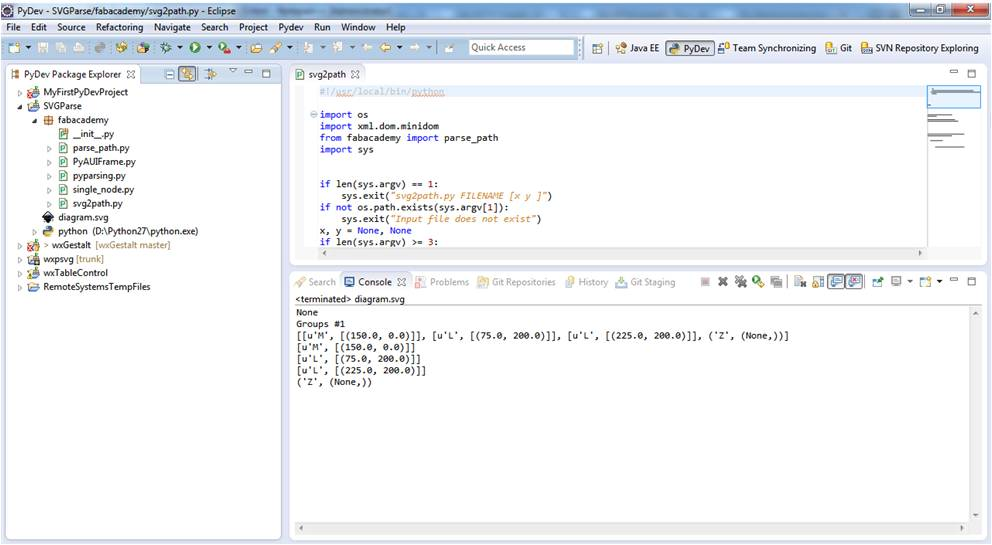### Mechanical Design, Machine DesignExercise...For this week assignment we have to work in group and make a machine that does something. We have to design all the machine and automate it.

We have done a preliminary study of gestalt libraries and together in opendot fab lab made some test with cardboard and axis in order to understand how it works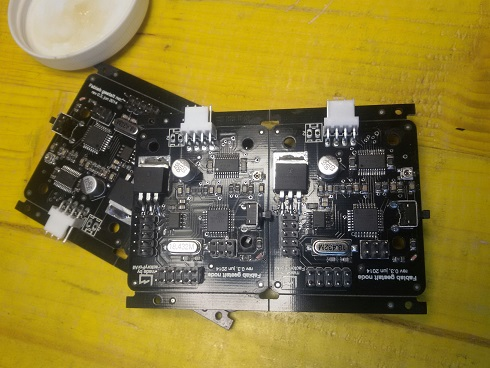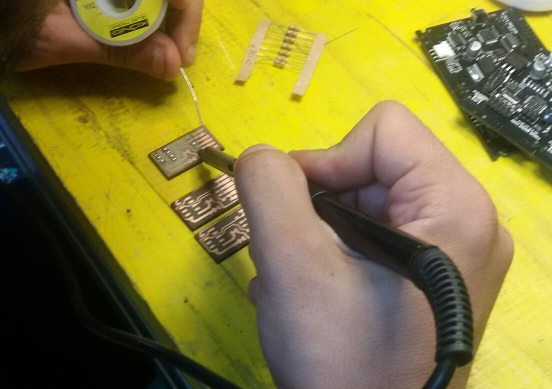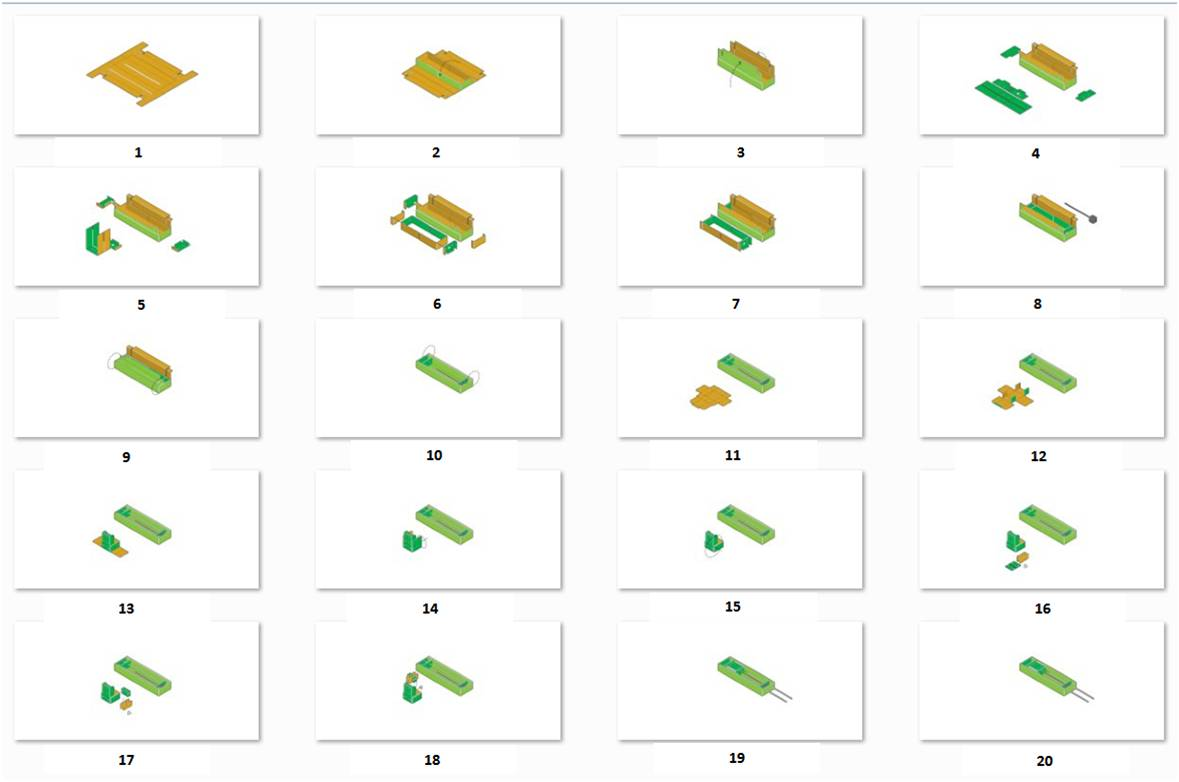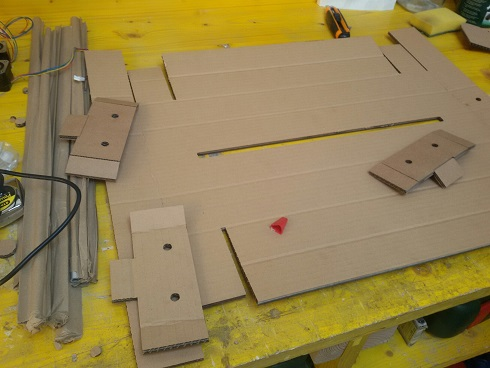After this experimentation, Daniele Ingrassia, Youssef Bouali, Claudio Pecere and me were in charge of the development of the software for the machines. The language used is Python. In particular I was involved on SVG understanding and the develop of a SVG parser, needed manly for the XY plotter "Zen Garden" proposed by Saverio Silli.

To do this I created an Eclipse project by mean of PyDev plugin named "SVGParse". Successively this project has been integrate in Daniele MTM PyDev Project.

In particular the project contains:

parse_path.py the parser itself. In particular to use the method parse_path.get_path_commands
svg2path.py contains an example of how to read the xml file of SVG and pass it to the above method
PyAUIFrame.py an example interface wxPython with a file picker to select the SVG and the event handler that calls the method above .

After a first attempt with the wxPython object wx.html.HtmlWindow I realized that the elementary browser cannot render svg constructs so at the moment the preview is not implemented yet. A future implementation can be realazed by using CAIRO libraries or pyWxSVG ones.

The parse use a Path object able to manage the following SVG commands:

```#The  element is used to define a path.
#The following commands are available for path data:

#M = moveto
#L = lineto
#H = horizontal lineto
#V = vertical lineto
#C = curveto
#S = smooth curveto
#T = smooth quadratic Bezier curveto
#A = elliptical Arc
#Z = closepath
```

The code svg2path.py takes as input an svg file ( svg2path.py FILENAME [x y ] x and y are option) and provides the array of SVG commands.

```[['M', [(150.0, 0.0)]], ['L', [(75.0, 200.0)]], ['L', [(225.0, 200.0)]], ('Z', (None,))]
```

The library takes only SVG pure

svg2path.py
```#!/usr/local/bin/python

import os
import xml.dom.minidom
import sys

if len(sys.argv) == 1:
sys.exit("svg2path.py FILENAME [x y ]")
if not os.path.exists(sys.argv):
sys.exit("Input file does not exist")
x, y = None, None
if len(sys.argv) >= 3:
x = float(sys.argv)
y = float(sys.argv)
print "eeeee"

svg_file = xml.dom.minidom.parse(sys.argv)
svg = svg_file.getElementsByTagName('svg')

raw_width = float(svg.getAttribute('width'))
raw_height = float(svg.getAttribute('height'))

print x
if (not(x,y==None) and  raw_width>x and raw_height>y):
sys.exit("dimension")
#width_ratio = x and (x / raw_width) or 1
#height_ratio = y and (y / raw_height) or 1

pathCommands=[]
elements = svg.getElementsByTagName('g')
print "Groups #"+str(elements.__len__())
for e in elements:
paths = []
if e.nodeName == 'g':
for path in e.getElementsByTagName('path'):
#print parse_path.path.parseString(path.getAttribute('d'))
pathCommands = parse_path.get_path_commands(path.getAttribute('d'))
print pathCommands
for command in pathCommands:
print  command

```